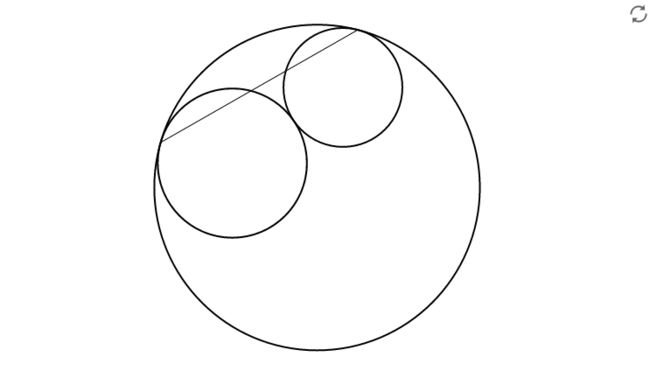# Allegro

Geometry Level 5The diagram shows three circles that are tangent to each other.
The diameters of the circles are $2$, $3$, and $6$.
The endpoints of the chord are the points of tangency of the smaller circles and the largest circle.
Find the length of the chord.

×